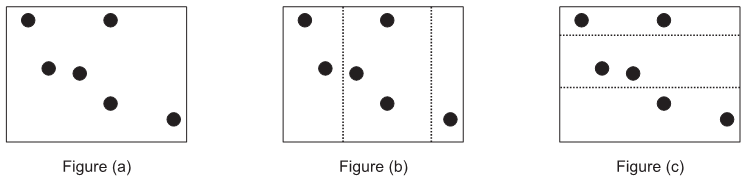Online JudgeProblem SetAuthorsOnline ContestsUser
Web Board
F.A.Qs
Statistical Charts
Problems
Submit Problem
Online Status
Prob.ID:
Register
Authors ranklist
Current Contest
Past Contests
Scheduled Contests
Award Contest
Register
Language:
Land Division
 Time Limit: 1000MS Memory Limit: 65536K Total Submissions: 284 Accepted: 105

Description

The king of the Far, Far Away Kingdom has passed-away and the kingdom must be split amongst his K sons. The kingdom, which can be drawn on a rectangular map, consists of N cities. To divide the land, they will draw K - 1 straight segments on the map, all of them parallel to either the vertical or the horizontal axis of the map. This divides the map into exactly K rectangles, all having equal heights (if the dividing lines where vertical), or equal widths (if the dividing lines where horizontal). No segment should pass through any of the cities. Each son will then be assigned one random region out of the K regions and the cities inside that region will be his share.
Of course, they want the division to be as fair as possible: theoretically, in the fairest division, each son should get N/K cities (we’ll call this value the baseline), but since the baseline isn’t always a whole number, each of the sons wants to be as close as possible to the baseline. We will calculate the unfairness of each son as the absolute difference between the number of cities assigned to him and the baseline. The fairest division is the one that minimizes the average unfairness of all the sons.Consider the example above with 3 sons and 6 cities (so the baseline is 6/3 = 2.0) Figure (a) is the original map. Figure (b) shows a non-optimal division (the dashed lines are the 2 dividing lines.) In this case, the middle region contains 3 cities (unfairness of |3 - 2| = 1), the left region contains 1 city (unfairness of |1 - 2| = 1), while the right region contains 2 cities (perfectly fair, unfairness of 0), so the average unfairness is 2/3.
Figure (c) on the right shows the optimal division since all three regions contain the same number of cities for an average unfairness of 0.
Write a program to determine the fairest land division for a given kingdom.

Input

Your program will be tested on one or more test cases. Each test case is described on N +1 lines. The first line of each test case specifies two positive integers: (N ≤ 100, 000) and (K ≤ 10) where N is the number of cities and K is number of children. Note that K ≤ N.
N lines follows, each describing the coordinates of a city by specifying two integers (x, y) where 0 ≤ x, y ≤ 100, 000. Since coordinates are rounded to the nearest integer, more than one city could have the exact same coordinate on the map. You may assume that the map of the kingdom is any rectangle that contains all of the given points (although such information is not needed by the program.) Note also that while all cities lie on integer coordinates, the dividing lines need not be.
The last line of the input file contains two zeros.

Output

For each test case, print the following line:
k. A/B
Where k is the test case number (starting at one,) and A/B is the minimum average that could be obtained. A/B should be an irreducible fraction. Let B=1 when the result is a whole number.

Sample Input

```6 3
0 4
1 3
2 3
3 1
4 4
5 0
4 3
0 0
0 1
1 1
1 0
0 0```

Sample Output

```1. 0/1
2. 8/9```

Source

[Submit]   [Go Back]   [Status]   [Discuss]Home PageGo BackTo top